# How do Dirichlet and Neumann boundary conditions affect Finite Element Methods variational formulations?

To solve a classical second-order differential problem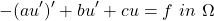with FEM, we first need to derive its weak formulation. This is achieved by multiplying the equation by a test function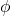and then integrating by parts to get rid of second order derivatives:

(1)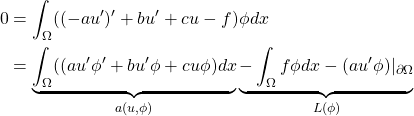A typical FEM problem then reads like: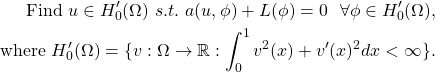What is the difference between imposing Dirichlet boundary conditions (ex.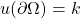) and Neumann ones (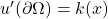) from a math perspective? Dirichlet conditions go into the definition of the space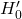, while Neumann conditions do not. Neumann conditions only affect the variational problem formulation straight away.

For example, in one dimension, adding the Dirichlet condition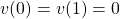results in the function space change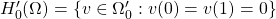. With this condition, the boundary term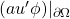would also zero out in the variational problem. because the test functionbelongs to.

On the other hand, by adding the Neumann condition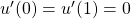, the spacedoes not change, even though the boundary term vanishes from the variational problem in the same way as the for the Dirichlet condition. However, that term goes to zero not because of the test function anymore, but because of the value of the derivative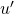. If the Neumann condition had specified a different value, such as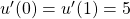, then the boundary term would not zero out!

In other words, Dirichlet conditions have the effect of further constraining the solution function space, while Neumann conditions only affect the equations.